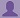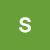SHARE
HELP

To start with, Sr2Jr’s first step is to reduce the expenses related to education. To achieve this goal Sr2Jr organized the textbook’s question and answers. Sr2Jr is community based and need your support to fill the question and answers. The question and answers posted will be available free of cost to all.# Absolute Java

Authors:
Walter Savitch ,kenrick Mock
Exercise:
Programming Projects
Chapter:
Flow Of Control
Edition:
5
ISBN:
9780132830317
Question:
1
 Previous Next

###Question

(This is a version of Programming Project 2.1 from Chapter 2.) The Babylonian algorithm to compute the square root of a positive number n is as follows:

1. Make a guess at the answer (you can pick n/2 as your initial guess).

2. Compute r = n / guess.

3. Set guess = (guess +r) / 2.

4. Go back to step 2 until the last two guess values are within 1% of each

other.

Write a program that inputs a double for n , iterates through the Babylonian algorithm until the guess is within 1% of the previous guess, and outputs the answer as a double to two decimal places. Your answer should be accurate even for large values of n.

###Answer

Program:

``````// BabylonianAlgorithm.java
import java.util.Scanner;
public class BabylonianAlgorithm
{
public static void main(String[] args)
{
Scanner keyboard = new Scanner(System.in);

double n;
double guess;
double r;
double previousGuess;
double diff;

System.out.print("Enter a value for n: ");
n = keyboard.nextDouble();

guess = n / 2;

do
{
r = n / guess;
previousGuess = guess;
guess = (guess + r) / 2;
diff = 	(previousGuess - guess) / (0.01 * previousGuess);
}while(diff > 0);

System.out.printf(
"The square root of %.2f is approximately: %.2f\n", n, guess);

keyboard.close();
}
}``````

Output:

``````Enter a value for n: 225
The square root of 225.00 is approximately: 15.00``````

###sinaaa

import java.util.Scanner;

public class Review1 {

public static void main(String[] args) {
Scanner keyboard = new Scanner(System.in);
System.out.print("Enter a value for n: ");
double n = keyboard.nextDouble();
double guess = n/2;
double r, pGuess, percentage;

for(int i=0; i>=0; i++)
{
r = n/guess;
pGuess = guess;
guess = (guess+r) /2;
percentage = ((pGuess-guess)/((pGuess+guess)/2)*100);
System.out.printf("%.2f\n", percentage);
if (percentage<0.1)
break;

}
System.out.printf("The square root of %.1f is approximately: %.5f\n", n, guess);
keyboard.close();
}

}

###sinaaa

import java.util.Scanner;

public class Review1 {

public static void main(String[] args) {
Scanner keyboard = new Scanner(System.in);
System.out.print("Enter a value for n: ");
double n = keyboard.nextDouble();
double guess = n/2;
double r, pGuess, percentage;

for(int i=0; i>=0; i++)
{
r = n/guess;
pGuess = guess;
guess = (guess+r) /2;
percentage = ((pGuess-guess)/((pGuess+guess)/2)*100);
System.out.printf("%.2f\n", percentage);
if (percentage<0.1)
break;

}
System.out.printf("The square root of %.1f is approximately: %.5f\n", n, guess);
keyboard.close();
}

}

Post the discussion to improve the above solution.